# Free vibrations

In the introductory tutorial in this series we considered a free vibrating translational spring and mass system with one degree of freedom as shown in Figure 1 below. Mass m connected to a spring with spring constant k is free to move on a horizontal frictionless plane from the static equilibrium position x = 0.  The diagram shows the force Fs exerted by the spring on mass m in extension (i) and compression (ii).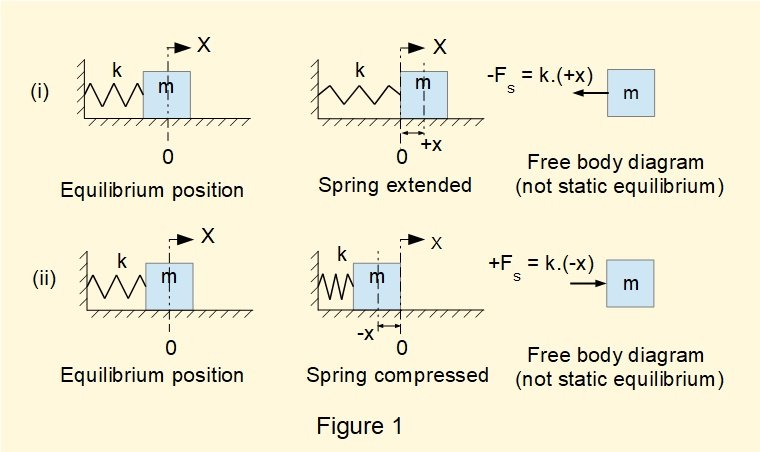From dynamic* free body diagrams we obtained the following equation of motion in terms of displacement x and time t noting that this equation is an expression of simple harmonic motion (SHM).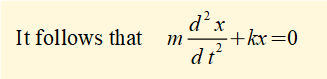*  showing forces producing acceleration of mass m, not static equilibrium

We now find a function x(t) that is a general solution to this equation.  If you are not familiar with solving second-order homogeneous linear differential equations using the characteristic equation method refer to the  maths tutorial.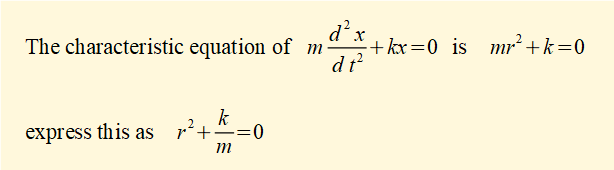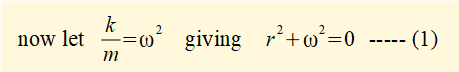Note that k has dimensions ML / T2L  (force / displacement).  Thus ω2 and ω = √(k / m)  have dimensions respectively 1 / T2 and 1 /  T.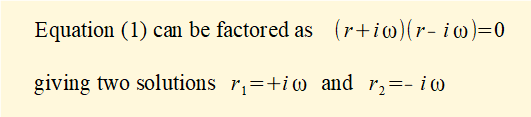From the maths tutorial we see that two complex conjugate roots of the characteristic equation expressed as:

r1 =( α + iβ)  and  r2 =  (α - iβ)  give the following general solution  for x = f(t):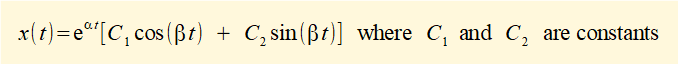In the case above   r1, 2 = ± iω      Thus α = 0  and   β = ω  giving the general solution for the equation of motion as: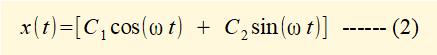It is conventional to replace constants C1 and C2 with A and B giving:

x(t) = [Acos(ωt) + Bsin(ωt)] ---------- (3)

We now find a particular solution for (3) using the following initial conditions at time t = 0 :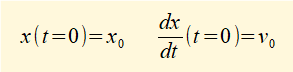This means that at time t = 0 the extension of the spring is x0 and the mass is given initial velocity v0

Inserting initial condition x0 into equation (3) at time t = 0 gives:

x0 = Acos(ωt) + Bsin(ωt) = (A).(1) + (B).(0) = A   thus A = x0v0 = -ωA.sin(ωt) + ωB.cos(ωt) = -ωA.(0) + ωB.(1)     thus B = (v0 / ω)

x(t) can now be expressed in terms of initial conditions x0 and v0 as;

x(t) = x0cos(ωt) + (v0 / ω) sin(ωt) ------- (4)

It is convenient to express the equation of motion in terms only of cos(ωt) using the following identity:

Acos(ωt) + Bsin(ωt) = Rcos(ωt -  φ)     where R = √(A2 +B2) and  φ = tan-1 B /A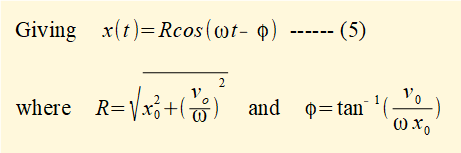R is the maximum absolute value (or amplitude) of x(t).

ω represents the angular frequency of the SHM in radians/sec, called the natural frequency of the system denoted by ωnωn / 2π is the natural frequency measured in Hertz.  The period T of one cycle is the reciprocal of the frequency = 2π / ωn .

Figure 2 below plots displacement x(t) = R.cos(ωt  - φ) against time t for a spring and mass system with the following parameters:

mass m = 1 kg    spring constant k = 10 N/m     initial displacement x0 = 0.1 m     initial velocity v0 =  1 m/s.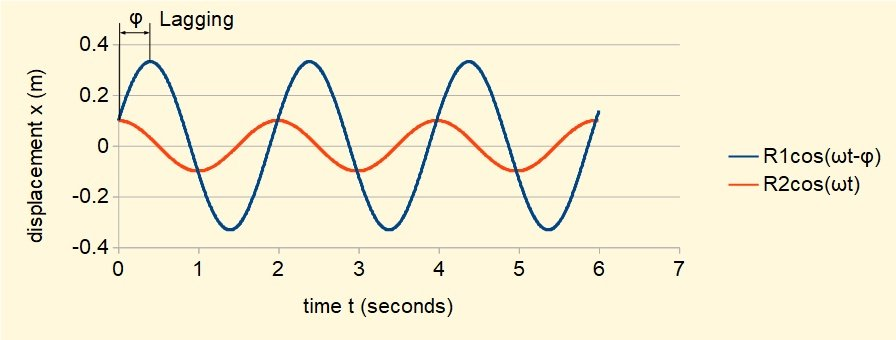In this example ωn = √(k / m) = √(10 / 1) = √((10) rad/sec equivalent to  (ωn / 2π)  = 0.503 Hz..  Period T = (2π / ωn ) = (1 / 0.503) = 1.99 s.  Amplitude R = 0.332 m.

Figure 2 also plots x(t) = R.cos(ωt) which represents SHM with initial conditions x0 = R and v0 = 0.  Note that amplitude and frequency of both motions are identical.  The phase angle φ between the two oscillations in this example = 72.5° with R.cos(ωt - φ)  lagging R.cos(ωt)

In the next tutorial we consider free vibrations with damping.

I welcome feedback at:

### Tutorials - mechanical vibrations

###### Forced vibrations with damping

Alistair's tutorials 2021